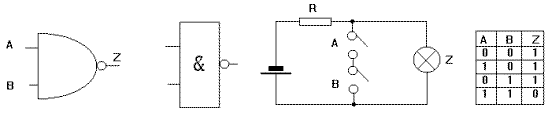Home > Electronic Tutorials > Digital Circuits Tutorial > NAND Gates Tutorial

Digital Circuits - Electronic Tutorials

# NAND Gates Tutorial

The Nand gate has two or more inputs and one output.

The output voltage goes low only when all input voltages are high.

In the switch diagram the lamp goes out  when A and B are operated. (A short circuit is placed across the lamp).

In the truth table Z = 0  when A and B = 1.

_
The Boolean expression is A.B = Z which translated says, A and B high makes Z low.
_
Z (called Z bar) means Z is low.US symbol UK symbol switches simulating NAND truth table